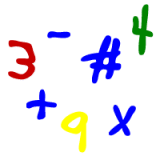Parents and Teachers: Support Ducksters by following us onor.

# Kids MathKids Math Subjects

 Practice Problems Addition One Digit Addition Practice Questions Two Digit Addition Practice Questions Three Digit Addition Practice Questions Addition Math Game Subtraction One Digit Subtraction Practice Questions Three Digit Subtraction Practice Questions Subtraction Math Game Multiplication One Digit Multiplication Practice Questions Three Digit Multiplication Practice Questions Multiplication Times Tables Game Division One Digit Division Practice Questions Two Digit Division Practice Questions Division Math Game Word Problems One and two Digit Word Problems Math Glossaries and Terms Algebra glossary Angles glossary Figures and Shapes glossary Fractions glossary Graphs and lines glossary Measurements glossary Mathematical operations glossary Probability and statistics glossary Types of numbers glossary Units of measurements glossary Kids Math Games See all Math Games The Kids Math Challenge game allows students to set up the game to their learning level. It can be used to practice addition, subtraction, multiplication, and division. The game can be set up for just one of these math subjects or all four. The Kids Times Table game allows students to practice one multiplier at a time or 1-12 all at once. Fast Math for Kids helps develop speed in the basic math subjects.

Back to Kids Study PageFollow us onor